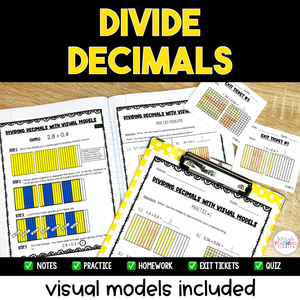# Dividing Decimals - Visual Models Included - Printable

• \$12.50
Unit price per

Use visual models along with the standard algorithm to quickly and effectively teach your students how to divide decimals with this comprehensive resource pack.

With 6 mini-lessons, (5 using the standard algorithm, and one with visual models) you will have plenty of resources to teach your student how to solve every type of decimal division problem.

Using visual models, students will have a more hands-on approach to “see” what it looks like when dividing decimals using tenths and hundredths in addition to the various types of problems with the standard algorithm.  It’s great for kinesthetic learners as well as students that may struggle with memorizing algorithms.

This resource is complete, comprehensive, and easy to follow with a clear format.  It is low-prep and easy to assign to a sub and contains engaging activities.  If your students are struggling conceptually, this resource is for you!

Standards Included:  5.NBT.7

This resource is also part of my 5th Grade Math Year-Long Curriculum Bundle.

How Can You Use This?

Introduce each skill with the detailed set of notes and sample problems.  I suggest doing this in a whole group setting and pulling the PDF up on a Smartboard so students can annotate over the models.

Gradually release students to practice independently using the practice pages.  2 Homework pages are also included for reinforcement at home.  These can also be used as additional classwork practice.  Check for understanding and mastery after teaching each skill using the exit tickets.

When all skills have been taught, provide additional practice and review using the set of task cards, then assess with the final quiz when you feel students are ready.

## What You Get

The 6 Mini-Lessons Included:

• Dividing Decimals by Whole Numbers
• Dividing Whole Numbers by Decimals
• Dividing Decimals by Decimals (with the same # of decimal places in the dividend and divisor)
• Dividing Decimals by Decimals (with a different # of decimal places in the dividend and divisor)
• Dividing Decimals by Decimals in which you have to annex a zero in the dividend
• Dividing Decimals by Decimals using a visual model

What You’ll Get in EACH of the 3 mini-lessons:

• Detailed Notes
• Sample Problems
• 2 practice pages
• 2 Homework Pages
• Exit Tickets (2-4 *The amount varies depending on the mini-lesson*)

You’ll Also Get: